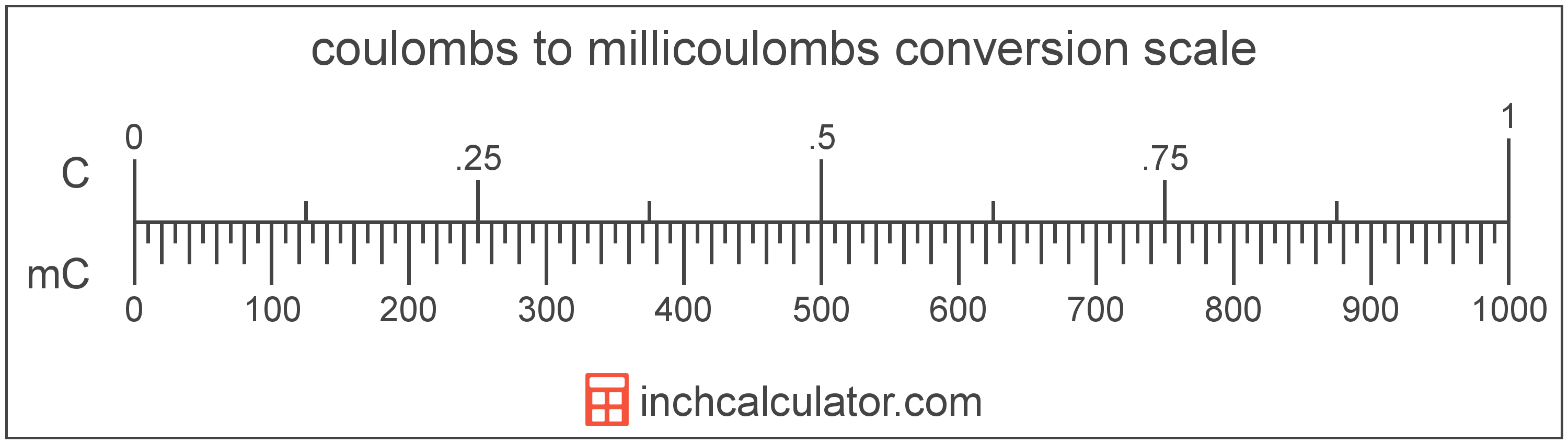# Coulombs to Millicoulombs Conversion

Enter the electric charge in coulombs below to get the value converted to millicoulombs.

Results in Millicoulombs:1 C = 1,000 mC

## How to Convert Coulombs to Millicoulombs

To convert a coulomb measurement to a millicoulomb measurement, multiply the electric charge by the conversion ratio.

Since one coulomb is equal to 1,000 millicoulombs, you can use this simple formula to convert:

millicoulombs = coulombs × 1,000

The electric charge in millicoulombs is equal to the coulombs multiplied by 1,000.

For example, here's how to convert 5 coulombs to millicoulombs using the formula above.
5 C = (5 × 1,000) = 5,000 mC### How Many Millicoulombs are in a Coulomb?

There are 1,000 millicoulombs in a coulomb, which is why we use this value in the formula above.

1 C = 1,000 mC

## Coulombs

One coulomb is the electric charge equal to one ampere of current over one second.

The coulomb can be expressed as QC = IA × ts

The charge in coulombs is equal to the current in amperes times the time in seconds.

The coulomb is the SI derived unit for electric charge in the metric system. Coulombs can be abbreviated as C; for example, 1 coulomb can be written as 1 C.

## Millicoulombs

The millicoulomb is 1/1,000 of a coulomb, which is the electric charge equal to one ampere of current over one second.

The millicoulomb is a multiple of the coulomb, which is the SI derived unit for electric charge. In the metric system, "milli" is the prefix for 10-3. Millicoulombs can be abbreviated as mC; for example, 1 millicoulomb can be written as 1 mC.

## Coulomb to Millicoulomb Conversion Table

Coulomb measurements converted to millicoulombs
Coulombs Millicoulombs
0.001 C 1 mC
0.002 C 2 mC
0.003 C 3 mC
0.004 C 4 mC
0.005 C 5 mC
0.006 C 6 mC
0.007 C 7 mC
0.008 C 8 mC
0.009 C 9 mC
0.01 C 10 mC
0.02 C 20 mC
0.03 C 30 mC
0.04 C 40 mC
0.05 C 50 mC
0.06 C 60 mC
0.07 C 70 mC
0.08 C 80 mC
0.09 C 90 mC
0.1 C 100 mC
0.2 C 200 mC
0.3 C 300 mC
0.4 C 400 mC
0.5 C 500 mC
0.6 C 600 mC
0.7 C 700 mC
0.8 C 800 mC
0.9 C 900 mC
1 C 1,000 mC

## References

1. International Bureau of Weights and Measures, The International System of Units, 9th Edition, 2019, https://www.bipm.org/documents/20126/41483022/SI-Brochure-9-EN.pdf